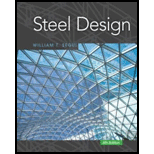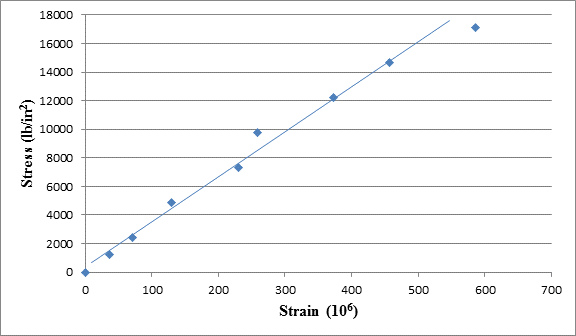Chapter 1, Problem 1.5.3P### Steel Design (Activate Learning wi...

6th Edition
Segui + 1 other
ISBN: 9781337094740

#### Solutions

Chapter
Section### Steel Design (Activate Learning wi...

6th Edition
Segui + 1 other
ISBN: 9781337094740
Textbook Problem

# A tensile test was performed on a metal specimen having a circular cross section with a diameter 0. 510 inch. For each increment of load applied, the strain was directly determined by means of a strain gage attached to the specimen. The results are, shown in Table: 1.5.1.a. Prepare a table of stress and strain.b. Plot these data to obtain a stress-strain curve. Do not connect the data points; draw a best-fit straight line through them.c. Determine the modulus of elasticity as the slope of the best-fit line.To determine

(a)

The stress and strain values.

Explanation

Given:

The diameter of metal specimen is 0.510inch.

Concept Used:

Write the equation of tensile stress.

f=PA      ...... (I)

Here, tensile stress is f, load is P and the cross-sectional area is A.

Calculation:

Calculate the cross-sectional area.

A=π4×d2      ...... (II).

Here, the diameter of metal specimen is d.

Substitute 0.510inch for d in Equation (II).

A=π4×(0.510inch)2=0.8171inch24=0.2042inch2

Calculate the stress value corresponding to load and the cross-sectional area.

The following table shows the stress value.

 LoadP(lb) Strain (×10−6) Stress f=PA Stress (lb/in2) 0 0 f=00.2042 0 250 37.1 f=2500.2042 1224.28 500 70.3 f=5000.2042 2448.57 1000 129.1 f=10000.2042 4897.15 1500 230
To determine

(b)

The plot of the stress-strain curve.

To determine

(c)

The modulus of elasticity from the slope of best-fit line.

### Still sussing out bartleby?

Check out a sample textbook solution.

See a sample solution

#### The Solution to Your Study Problems

Bartleby provides explanations to thousands of textbook problems written by our experts, many with advanced degrees!

Get Started

## Additional Engineering Solutions

#### Find more solutions based on key concepts